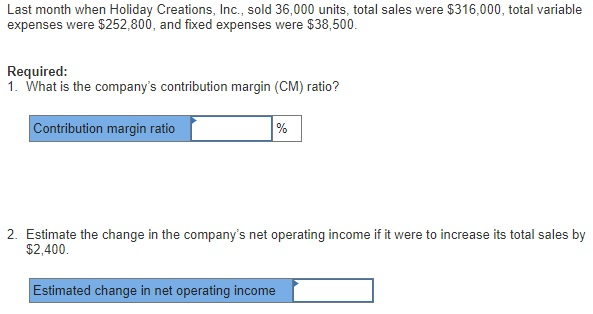# Question & Answer: Last month when Holiday Creations, Inc., sold 36,000 units, total sales were \$316,000, total variable expenses were \$2…..Last month when Holiday Creations, Inc., sold 36,000 units, total sales were \$316,000, total variable expenses were \$252, 800, and fixed expenses were \$38, 500. Required: What is the company’s contribution margin (CM) ratio? Estimate the change in the company’s net operating income if it were to increase its total sales by \$2, 400.

Don't use plagiarized sources. Get Your Custom Essay on
Question & Answer: Last month when Holiday Creations, Inc., sold 36,000 units, total sales were \$316,000, total variable expenses were \$2…..
GET AN ESSAY WRITTEN FOR YOU FROM AS LOW AS \$13/PAGE

1. Contribution margin = Sales – Variable expenses = \$316,000-\$252,800 = \$63,200

Contribution margin ratio = Contribution margin/Sales = \$63,200/\$316,000 = 0.20 = 20%

2.

Change in net operating income = Change in sales*Contribution margin ratio

Increase in net operating income = \$2,400*20% = \$480